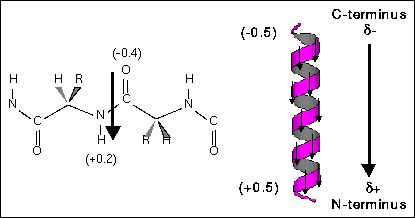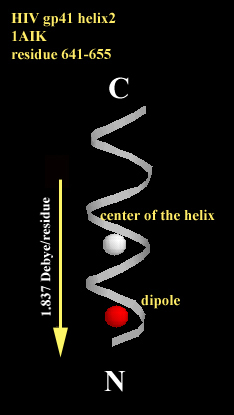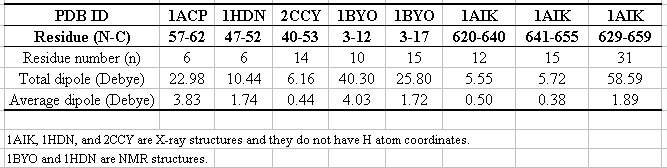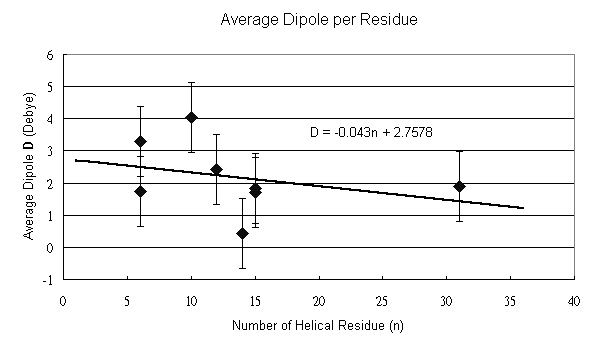# Helix Dipole

Introduction
Helix macrodipole is a cumulative effect of each residue backbone unit dipole contribution. Because helical peptides have periodic and well ordered structures, every backbone dipole vector resulted from partial charge of each atom points to approximately the same direction, as show in Fig.1, and results a macrodipole from C-terminus to N-terminus. Since this is an cumulative effect, the macrodipole (D) should be depedent to the length of the helix, i.e., the residue number (n). We can use the coordinate files in the protein data bank (PDB) to calculate the helix dipole and examine the dependence of the length.Fig. 1 Each peptide bond contributes a dipole vector toward the N-terminus, and the ensamble of the whole helix gives a helix macrodipole.

Method
First of all, select one coordinate file, which contains at least one helix, from the protein data bank. Usually, the coordinate file description has notations about its secondary structure (starting and ending residue numbers), so that we can only select the helical region as our target. The center of the helix (xo, yo, zo) is determined by the average of all backbone atom coordinates in each dimension ( Sri/Si ) and the separation of each dipole partial charge d is then calculated by the difference of each atom and the center of the helix ri-ro=(xi-xo, yi-yo, zi-zo). The macrodipole (D) is then determined by sum of the dipole contribution of each backbone atom Di=(ri-ro)*di . Theoretically, the average dipole (D/n) of each residue should be independent to the length of the helix.

Result
The center of the helix and the helix dipole are calculated and shown in spheres in Fig. 2a and Fig. 2b. The magnitude of the macrodipole is proportional to the length of the helix.2a2b

Fig. 2  The center of the helix is shown as white shpere and the helix dipole is shown as red shpere (it is shown in the unit of [unit charge*angstrum]). The dipole of each directs toward the N-terminus along the helical axis. 2a. The helix of 15 amino acids is a fragment of HIV coat protein gp41 from residue 641 to residue 655. the average dipole per residue is 1.837 Debye/residue. 2b. The helix of 10 amino acids is a fragment of hepatitus delta antigen from residue 3 to residue 12. the average dipole per residue is 4.031 Debye/residue.

Table 1 shows the results of different protein and peptide structures. The average dipole per residue is 2.17 (+/-)1.09 Debye, which is of the same order of the given number in the text book (3.73 Debye).Table 1  The NMR structures contain N, Ca, C, O, H and Ha coordinates, but X-ray structures do not  have hydrogen atom coordinates. This may differ the contribution of each backbone unit.

The relationship between the length and the dipole is depicted in Fig. 3. From the result, we can find a decreasing tendency as the number of the residue increases. However, the acumulative effect varies in different helice and different length. As shown in Table 1, calculations of 1AIK with different residues give different average dipole, whereas the same helix length of 6 a. a. in 1ACP and 1HDN, and of 15 a. a. in 1BYO and 1AIK give different averge dipole. This inconsistency may be explained by the effect different environments and side chains. The bending and twisting of long helice can also affect the helix dipole.Fig. 3  The data points are fitted by the given linear equation D=-0.043n+2.758 (Debye/n) with a error bar of (+/-)1.09 (Debye/n), which is calculated from the eight data points. The negative slope indicates a decreasing tendency as the length of the helix increases.